This is in continuation of my article on getting started with measure theory. As a measure theory student, you may have gone through the trouble of selecting the best books on measure theory. I had the same troubles once. That’s why I have collected the 15 best measure theory books for graduate students.

Here is the list:

Photo Title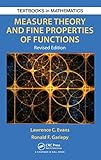Measure Theory and Fine Properties of Functions, Revised Edition (Textbooks in Mathematics)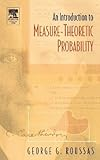An Introduction to Measure-theoretic Probability by George G. Roussas (2004-11-02)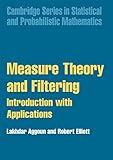Measure Theory and Filtering: Introduction and Applications (Cambridge Series in Statistical and Probabilistic Mathematics Book 15)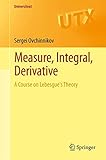Measure, Integral, Derivative: A Course on Lebesgue’s Theory (Universitext)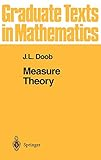Measure Theory (Graduate Texts in Mathematics, 143)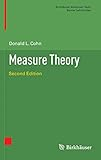Measure Theory: Second Edition (Birkhäuser Advanced Texts Basler Lehrbücher)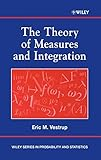The Theory of Measures and Integration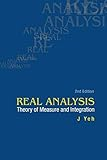Real Analysis: Theory Of Measure And Integration (2Nd Edition)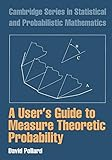A User’s Guide to Measure Theoretic Probability (Cambridge Series in Statistical and Probabilistic Mathematics)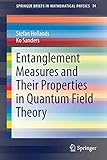Entanglement Measures and Their Properties in Quantum Field Theory (SpringerBriefs in Mathematical Physics, 34)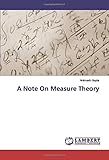A Note On Measure Theory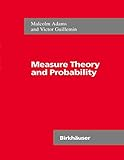Measure Theory and Probability (The Wadsworth & Brooks/Cole Mathematics Series)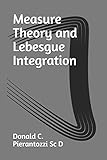Measure Theory and Lebesgue Integration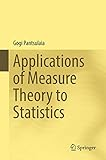Applications of Measure Theory to Statistics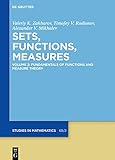Fundamentals of Functions and Measure Theory (De Gruyter Studies in Mathematics)

Let’s dive in detail.

## 1. Measure Theory and Fine Properties of Functions, Revised Edition (Lawrence Craig Evans, Ronald F. Gariepy)

Measure Theory and Fine Properties of Functions is notable for providing a thorough analysis of the core assertions of measure theory in n-dimensional Euclidean space. The importance of Hausdorff measure and capacity in characterizing the fine properties of functions and sets has been particularly focused on here. The main topics included in the book are Hausdorff measures, theorems and differentiation in Rn, a quick review of abstract measure theory, Sobolev functions, functions of bounded variation, area and coarea formulas for Lipschitz mappings, and change-of-variable formulas associated with the same.

Notably, this book features wholesome proofs of various key results that are not to be found in other books, such as Rademacher’s theorem, Besicovitch’s covering theorem, the precise structure of sets of finite perimeter, the precise structure of BV and Sobolev functions, and Aleksandrov’s theorem. In this revised edition, there are several improvements in format, clarity of exposition, and notation. You will also find various new sections describing the weak compactness criteria in L1, Young measure methods for weak convergence, and the π-λ theorem. The book’s topics have been chosen carefully, and proofs are concise but complete. Whether you are a professional mathematician or a graduate in applied or pure mathematics, this book is worth checking out.

## 2. An Introduction to Measure – Theoretic Probability (George G. Roussas)

An Introduction to Measure – Theoretic Probability, Second Edition beautifully teaches the basics of measure theoretic probability by taking a classical approach to the topic. In a succinct but comprehensive manner, the author provides most of the probabilistic tools needed by students pursuing advanced degrees in probability, statistics, and other similar areas. You don’t require any prior knowledge of measure theory to follow this edition. Every one of its topics is presented in rich detail, and it also has a chapter each on two cases of statistical estimation and the basics of ergodic theory.

The topics in this book include everything from the basic properties of a measure to modes of convergence of random variables and their relationships – standard convergence theorems, the integral of a random variable and its basic properties, the Lebesgue Decomposition T, the Hahn-Jordan Decomposition Theorem, ergodic theory, sequences of independent random variables, the theory of characteristic functions, conditional expectation and conditional probability. The book focuses a lot on the way probability is practically used in finance, statistical research, and various other areas.

The book also includes a large number of practical examples and lengthy exercises. All relevant proofs are presented in exquisite detail. You can access in-depth solutions to all the aforementioned exercises on the book companion site. To conclude, this book is an excellent resource for graduate students in mathematics, electrical and computer engineering, statistics, and various other information sciences. It is also helpful for students in mathematical economics or finance in the departments of economics.

## 3. Measure Theory and Filtering (Lakhdar Aggoun)

This book is mainly intended for readers who are not involved with the field of statistics. It provides a concise introduction to Stochastic calculus, stochastic processes, and measure theory. It also serves as an ideal user’s guide to filtering and includes plenty of useful exercises for students. It is an excellent resource for signal processing researchers, engineers, and anyone interested in practical implementation of filtering techniques (especially the Kalman filter). It features three different chapters dealing with applications arising in population modelling, genetics, and finance.

## 4. Measure, Integral, Derivative: A Course on Lebesgue’s Theory (Sergei Ovchinnikov)

Basically, this classroom-tested book has been written to serve as a one-semester course in Lebesgue’s theory. It features more than 180 exercises and opts for an elementary approach to the topic. Thus, it is readily accessible to both undergraduate and lower-graduate-level students. The only prerequisite for this book is a course in elementary real analysis. The three main topics that the book focuses on are measure, differentiation, and integration. An introductory chapter has also been included for helping the student bridge the gap between their previous knowledge and what the book has to offer.

For making the proofs of relatively difficult results more accessible to students, they have been divided into smaller steps. Except a handful of relatively simple statements, all the results given here have been proven. The book’s presentation is largely elementary, with Dini’s derivatives not being used in differentiation and σ-algebras not being used in the chapter on measure theory. That said, you will find all the central ideas of Lebesgue’s theory in the text.

## 5. Measure Theory (J.L. Doob)

Notably, this book accepts probability as an integral part of measure theory. Thus, it features a number of examples taken from probability, along with probabilistic concepts like Markov processes and independence. A significant amount of focus has been given to the role of algebras and the metric defining the distance between sets, because the measure of their symmetric difference is exploited much more than usual.

## 6. Measure Theory, Second Edition (Donald L. Cohn)

This textbook, which serves as a self-contained introduction to measure theory, comes with a detailed treatment on locally compact Hausdorff spaces, Haar measures on locally compact groups, and the Borel and analytic subsets of Polish spaces. The second edition of Measure Theory by Donald L. Cohn also features a chapter on measure-theoretic probability theory, along with concise explanations of the Daniell integral, the existence of liftings, the Banach-Tarski paradox, and the Henstock-Kurzweil integral.

This book offers an excellent background for study in both probability theory and functional analysis. It is a great resource for advanced undergraduate and graduate students in mathematics. The only prerequisites for this textbook are basic courses in analysis and point-set topology. The appendices have a detailed review of essential background material as well.

## 7. The Theory of Measures and Integration (Eric M. Vestrup)

The Theory of Measures and Integration by Eric Vestrup provides a well-organized and accessible survey of the basic topics of measure theory for both students and researchers in physics, statistics, and mathematics. You need to have elementary knowledge of measure theory and similar subjects in order to properly understand and appreciate advanced mathematical statistics, analysis, and advanced probability. This excellent book sheds light on the fundamental ideas of the subject and offers a useful theoretical background and solid foundation for further studies.

The book presents the prominent results of classical measure and integration theory in Eric Vestrup’s clear and measured writing style which is user-friendly and evenly paced. It also features comprehensive discussions of extensions, the Risesz representation theorem, the Hardy-Littlewood theorem, set-theoretic considerations, the structure of Borel and Lebesgue sets, and much more. Important chapters included in the text include:

• Measurable Functions
• The Lp spaces
• Products of Two Measure Spaces
• Arbitrary Products of Measure Spaces Sections

At the end of every chapter, there are exercises of varying difficulty levels – from relatively easy “finger exercises” to significant and independent points of interest. The tougher exercises come with comprehensive hints for showing students optional side paths in the subject, along with alternative methods of presenting mainstream topics. The book’s proofs and notations are particularly aimed towards readers who want all the details presented explicitly.

The Theory of Measures and Integration is a great choice for practicing researchers and both undergraduate and graduate students in physics, mathematics, and statistics. It is a worthy primary text for a real analysis sequence focusing on measure theory, and a useful background text for higher courses in statistics and probability.

## 8. Real Analysis: Theory of Measure and Integration (James J Yeh)

Real Analysis serves as a unified treatise of the theory of measure and integration. In the setting of a general measure space, the book defines every concept with crystal clear precision and presents every theorem with complete proof having all the necessary details. It also presents counter-examples to demonstrate that it isn’t possible to simply drop some conditions in the hypothesis of a theorem.

## 9. A User’s Guide to Measure Theoretic Probability (David Pollard)

A number of fields now feature rigorous probabilistic arguments, which have been built on the foundation of the measure theory introduced by Kolmogorov. Students from various disciplines like finance, econometrics, statistics, and biostatistics have been finding themselves in need of studying this theory in more depth. Published back in 2002, this book grew from a one-semester course to a mixed audience of both undergraduate and graduate students who haven’t studied measure theory previously.

The book mainly covers basic topics like conditioning, convergence in distribution, independence, Fourier transforms, and martingales. Also, there are a number of sections which deal with topics having a reputation of being more advanced, such as the isoperimetric inequality for Gaussian processes, option pricing via the equivalent martingale measure, and coupling and the KMT strong approximation. It is much more than a presentation of mathematical theory – it is a discussion of the reason for theory taking its present form. If you want to invoke rigorous probabilistic arguments and understand their meaning, then this is a good starting point for you.

## 10. Entanglement Measures and Their Properties in Quantum Field Theory (Stefan Hollands)

In this book, you will find a detailed presentation of entanglement measures in the general context of quantum field theory. It covers a wide range of models, and you will be able to properly take the localization of systems into account due to the use of fields. All the mathematical techniques needed here are introduced in a self-contained manner.

## 11. A Note on Measure Theory (Animesh Gupta)

This book aims to present a detailed description of modern abstract measure theory, along with some of its prominent applications. The first two volumes serve as introductory material for students who are well-versed in the concepts of real analysis, but require deeper knowledge of the same. The book focuses on the mathematical ideals involved, which are largely found in the details of the proofs. The book can be used both as an introduction to the subject and as a reference material.

To serve as an introductory work, the ideas of the early volumes have been limited to the ones truly vital for the development of the basic theorems. To serve as a reference material, these very ideas are expressed in their complete natural generality. The author has specifically avoided suggesting any needless restrictions in their applicability.

## 12. Measure Theory and Probability (Malcolm Adams, Victor Guillemin)

The writing style of this handy textbook is user-friendly to the topics covered by it. Thus, it is readily accessible to all kinds of students, regardless of their experience with the subject and knowledge of the same. The various informative exercises will prove to be greatly helpful to both instructors and students of statistical measure theoretic courses. A lot of the problems come with useful hints or solution outlines as well. Thus, this book should serve as a helpful reference material for students and professionals alike.

## 13. Measure Theory and Lebesgue Integration (Donald C. Pierantozzi)

The extension of the Riemann integral into a generalized partition set is undoubtedly content mainstream. Although this book is relatively short, it features highly concentrated material which is definitely not meant for light reading. The author assumes that the reader is well-versed in Riemann integration in the analysis, calculus, and advanced calculus, particularly in continuity and limits.

If you have a background in topology, it will prove to be quite useful while reading this book. The chapters in here are self-contained, and theory examples have been presented at important points. You should try to use supplementary material in working through some of the more detailed proofs of the more abstract theorems.

## 14. Applications of Measure Theory to Statistics (Gogi Pantsulaia)

This wonderful book by Gogi Pantsulaia puts powerful and reasonable mathematical senses in notions of subjectivity and objectivity for consistent estimations in a Polish group by using the concept of Haar null sets in the corresponding group. Basically, this is a refreshingly new approach which naturally divides the class of all consistent estimates of an unknown parameter in a Polish group into disjoint classes of objective and subjective estimates. With its help, the user can clarify some conjectures which arise in the criticism of null hypothesis significance testing.

By reading this book, you will also get acquainted with the theory of infinite-dimensional Monte Carlo integration, which has been recently developed for estimating the value of infinite-dimensional Riemann integrals over infinite-dimensional rectangles. It is a highly recommended book for both graduate students and researchers active in the fields of mathematical statistics, measure theory, and analysis.

## 15. Fundamentals of Functions and Measure Theory (Alexander V./Rodionov Mikhalev Timofey V./Zakharov Valeriy K.)

Fundamentals of Functions and Measure Theory is a detailed two-volume work dedicated to the most general foundations of mathematics. It revisits Hausdorff’s classic Set Theory, where the theory of functions and the set theory were described as the fundamental parts of mathematics in a way that left no requirement for references to other sources. Very similar to Hausdorff’s work, this book includes measure and integration theory as a basis of modern mathematics.